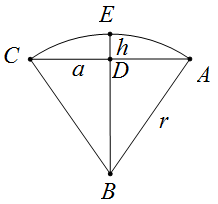# 球缺相关几何参数在线计算器

### App说明

r = AB；a = CD；h = ED$$V= \frac {πh}{6} (3a^2+h^2)$$

$$V= \frac {πh^2}{3} (3r-h)$$

$$F=π(2rh+a^2)$$

$$F=π(h^2+2a^2)$$

$$a^2=h(2r-h)$$

$$d^2=[2√[h(2r-h)]]^2$$

$$C=2πrh=π(a^2+h^2)$$

• r——球缺半径
• h——球缺的高
• a——平切圆半径
• d——平切圆直径

### 使用示例

h—球缺的高：4

r—球缺半径：7

Comment list ( 0 )

### 评价